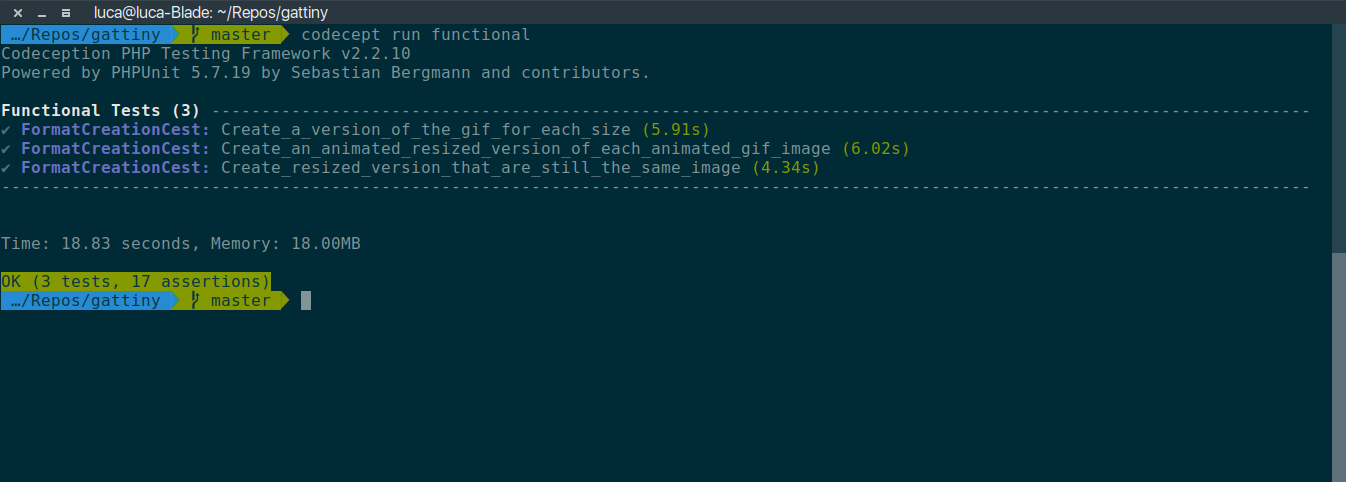# TDDing the “Gattiny” plugin – 03

Passing the last functional test to know it can be done.

## Part of a series

This is the third post in a series of posts chronicling my attempt to use test-driven development techniques to develop the “Gattiny” WordPress plugin.
The first post is probably a better starting point than this one.

## Where was I?

The last time I’ve worked on the code I had put in place 3 functional tests for the code; two of the tests were passing and one was failing.
At this stage of development I do not care about code style, standards, organization or practices: I just want to get the job done and pass the last test, this one:

// file tests/functional/FormatCreationCest.php

/**
* It should create resized version that are still the same image
*
* @test
*/
public function create_resized_version_that_are_still_the_same_image(FunctionalTester $I) {$I->loginAsAdmin();
$I->amOnAdminPage('media-new.php');$I->attachFile('input[name="async-upload"]', $this->gif);$I->click('input[name="html-upload"]');

$I->seeResponseCodeIs(200);$originalCoalesced = (new Imagick(codecept_data_dir($this->gif)))->coalesceImages();$I->amInPath($this->uploads); foreach ($this->sizeMap as $slug =>$size) {
if ($slug === 'full') { continue; }$suffix           = '' !== $size ? '-' .$size : '';
$file =$this->uploads . DIRECTORY_SEPARATOR . basename($this->gif, '.gif') .$suffix . '.gif';
$resizedCoalesced = (new Imagick($file))->coalesceImages();
list($w,$h) = explode('x', $size); // we test just the first frame$originalFrame = $originalCoalesced->getImage();$resizedFrame  = $resizedCoalesced->getImage(); if ($slug === 'thumbnail') {
$originalFrame->resizeImage($w, $h, Imagick::FILTER_BOX, 1, false);$resizedWidth  = $originalFrame->getImageWidth();$resizedHeight = $originalFrame->getImageHeight();$newWidth      = $resizedWidth / 2;$newHeight     = $resizedHeight / 2;$originalFrame->cropimage($newWidth,$newHeight, ($resizedWidth -$newWidth) / 2, ($resizedHeight -$newHeight) / 2);
$originalFrame->scaleimage($originalFrame->getImageWidth() * 4, $originalFrame->getImageHeight() * 4);$originalFrame->setImagePage($w,$h, 0, 0);
} else {
$originalFrame->resizeImage($w, $h, Imagick::FILTER_BOX, 1); }$comparison = $originalFrame->compareImages($resizedFrame, Imagick::METRIC_ROOTMEANSQUAREDERROR);
$I->assertTrue(0 <=$comparison && $comparison <= 0.1, "The {$slug} format image is not comparable");
}
}

When this test will pass I will stop writing functional tests and move to integration (one level down), and unit tests.
Once again writing the tests for the code provided me the code needed to pass them; specifically in an attempt to proerly handle cropping and scaling of the images for the purpose of comparison, see the last lines of the test method above, I came up with the code needed to handle that function on the backend.
The relevant code lives in the gattiny_GifEditor class: an extension of the WP_Image_Editor_Imagick class to handle and support of animated GIF images resizing;

// file src/GifEditor.php

<?php

class gattiny_GifEditor extends WP_Image_Editor_Imagick {

public static function test($args = []) { return !empty($args['mime_type']) && $args['mime_type'] === 'image/gif'; } public function multi_resize($sizes) {
$metadata = [];$original     = $this->image;$originalSize = $this->size;$testImage = $this->image->coalesceImages(); if ($testImage->count() === 1) {
return parent::multi_resize($sizes); } foreach ($sizes as $size =>$data) {
$resized =$this->resize($data['width'],$data['height'], $data['crop']);$duplicate = (($originalSize['width'] ==$data['width']) && ($originalSize['height'] ==$data['height']));

if (!is_wp_error($resized) && !$duplicate) {
$resized =$this->_save($this->image);$this->image->clear();
$this->image->destroy();$this->image = null;

if (!is_wp_error($resized) &&$resized) {
unset($resized['path']);$metadata[$size] =$resized;
}
}

$this->size =$originalSize;
$this->image =$original;
}

return $metadata; } public function resize($max_w, $max_h,$crop = false) {
$testImage =$this->image->coalesceImages();

if ($testImage->count() === 1) { return parent::resize($max_w, $max_h,$crop);
}

try {
$this->image =$testImage;

do {
if (!$crop) {$this->image->resizeImage($max_w, 0, Imagick::FILTER_BOX, 1, false);$this->update_size($max_w,$this->image->getImageHeight());
} else {
$this->image->resizeImage($max_w, $max_h, Imagick::FILTER_BOX, 1, false);$resizedWidth  = $this->image->getImageWidth();$resizedHeight = $this->image->getImageHeight();$newWidth  = $resizedWidth / 2;$newHeight = $resizedHeight / 2;$this->image->cropimage($newWidth,$newHeight, ($resizedWidth -$newWidth) / 2, ($resizedHeight -$newHeight) / 2);
$this->image->scaleimage($this->image->getImageWidth() * 4, $this->image->getImageHeight() * 4);$this->image->setImagePage($max_w,$max_h,0,0);
$this->update_size($max_w, $max_h); } } while ($this->image->nextImage());

return true;
} catch (Exception $e) { return new WP_Error('gattiny-resize-error', __('Gattiny generated an error: ', 'gattiny') .$e->getMessage());
}

}

public function _save(
$image,$filename = null,
$mime_type = null ) { if ($this->image->count() === 1) {
return parent::_save($image,$filename, $mime_type); } try {$this->image = $this->image->deconstructImages();$filename    = $this->generate_filename(null, null, 'gif');$this->image->writeImages($filename, true); /** This filter is documented in wp-includes/class-wp-image-editor-gd.php */ return [ 'path' =>$filename,
'file'      => wp_basename(apply_filters('image_make_intermediate_size', $filename)), 'width' =>$this->size['width'],
'height'    => $this->size['height'], 'mime-type' =>$mime_type,
];
} catch (Exception $e) { return new WP_Error('gattiny-save-error', __('Gattiny generated an error: ', 'gattiny') .$e->getMessage());
}

}
}

There is not much “exoticity” to it and the class is lacking from various points of view:

• tests are not checking how cropping works with non square images
• the code does not offer the same hooks and filters the WordPress class provides
• the code is not properly documented and the plugin has a juvenile structure

Still, in its lack of elegance, the code passes the tests:## What to do next?

The question of what to do next is one that’s better answered by another: “Which test should I write now?”.
When adopting a TDD approach this is really the only question that drives my development: the next test could be of any nature and covering as much code as the application or just a single class method.
Yet a new test means modeling a new feature, use case or API method and, thinking in this terms, it’s easy to find a new direction to move the code too.
Having those three functional tests in place makes sure that the plugin, so far, is behaving as intended and provides an “umbrella” under which I can refactor, move, simplify and redefine as much as I want.
With this is mind my next step will be to make sure that that plugin provides, in its functions, all the filters and actions the default WordPress class would provide.

## On GitHub

The code I’ve produced so far, in terms of code and tests for it, is on GitHub tagged post-03.

## Next

I will scaffold integration tests for the plugin and get the filtering in order; I will use the chance for some refactoring too.